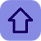## Top 20 Frequently asked questions in c programming

Posted by Tushar Bedekar

### Programs :

of the given number...
2. Write a program to check whether the given number is even or odd.
3. Write a program to swap two numbers using a temporary variable.
4. Write a program to swap two numbers without using a temporary variable.
5. Write a program to swap two numbers using bitwise operators.
6. Write a program to find the greatest of three numbers.
7. Write a program to find the greatest among ten numbers.
8. Write a program to check whether the given number is a prime.
9. Write a program to check whether the given number is a palindrome c number.
10.Write a program to check whether the given string is a palindrome .
11.Write a program to generate the Fibonacci series.
12.Write a program to print"Hello World"without using semicolon anywhere in the code.
13.Write a program to print a semicolon without using a semicolon anywhere in the code.
14.Write a program to compare two strings without using strcmp() function.
15.Write a program to concatenat e two strings without using strcat() function.
16.Write a program to delete a specified line from a text file.
17.Write a program to replace a specified line in a text file.
18.Write a program to find the number of lines in a text file..
19.Write a C program which asks the user for a number between 1 to 9 and shows the number. If the user
inputs a number out of the specified range, the program should show an error and prompt the user for a
valid input.
20.Write a program to display the multiplica tion table of a given number..

Note:-The solution will be published shortly stay connected with us.